# Lebesgue function

(diff) ← Older revision | Latest revision (diff) | Newer revision → (diff)

A function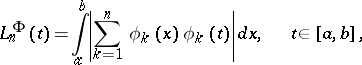where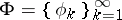is a given system of functions, orthonormal with respect to the Lebesgue measure on the interval,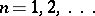. Lebesgue functions are defined similarly in the case when an orthonormal system is specified on an arbitrary measure space. One has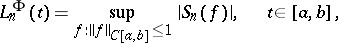where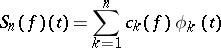is the-th partial sum of the Fourier series ofwith respect to. In the case whenis the trigonometric system, the Lebesgue functions are constant and reduce to the Lebesgue constants. They were introduced by H. Lebesgue.

How to Cite This Entry:
Lebesgue function. Encyclopedia of Mathematics. URL: http://encyclopediaofmath.org/index.php?title=Lebesgue_function&oldid=13866
This article was adapted from an original article by B.S. Kashin (originator), which appeared in Encyclopedia of Mathematics - ISBN 1402006098. See original article